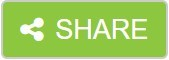# Overview

This course provides an overview of how to use IBM SPSS Modeler to predict a target field that describes numeric values. Students will be exposed to rule induction models such as CHAID and C&R Tree. They will also be introduced to traditional statistical models such as Linear Regression. Students are introduced to machine learning models, such as Neural Networks. Business use case examples include: predicting the length of subscription for newspapers, telecommunication, and job length, as well as predicting insurance claim amounts.

# Audience

IBM SPSS Modeler Analysts who have completed the Introduction to IBM SPSS Modeler and Data Mining course who want to become familiar with the modeling techniques available in IBM SPSS Modeler to predict a continuous target.

# Prerequisites

• Experience using IBM SPSS Modeler including familiarity with the Modeler environment, creating streams, reading data files, exploring data, setting the unit of analysis, combining datasets, deriving and reclassifying fields, and a basic knowledge of modeling. • Prior completion of Introduction to IBM SPSS Modeler and Data Science (v18.1.1) is recommended.

# Objective

1: Introduction to predictive models for continuous targets • List three modeling objectives • List two business questions that involve predicting continuous targets • Explain the concept of field measurement level and its implications for selecting a modeling technique • List three types of models to predict continuous targets • Determine the classification model to use

2: Building decision trees interactively • Explain how CHAID grows a tree • Explain how C&R Tree grows a tree • Build CHAID and C&R Tree models interactively • Evaluate models for continuous targets • Use the model nugget to score records

3: Building decision trees directly • Customize two options in the CHAID node • Customize two options in the C&R Tree node • List one difference between CHAID and C&R Tree

4. Using traditional statistical models • Explain key concepts for Linear • Customize options in the Linear node • Explain key concepts for Cox • Customize options in the Cox node

5: Using machine learning models • Explain key concepts for Neural Net • Customize one option in the Neural Net node

Show details

# Course Outline

1: Introduction to predicting continuous targets• List three modeling objectives• List two business questions that involve predicting continuous targets• Explain the concept of field measurement level and its implications for selecting a modeling technique• List three types of models to predict continuous targets• Determine the classification model to use2: Building decision trees interactively• Explain how CHAID grows a tree• Explain how C&R Tree grows a tree• Build CHAID and C&R Tree models interactively• Evaluate models for continuous targets• Use the model nugget to score records3: Building your tree directly• Explain the difference between CHAID and Exhaustive CHAID• Explain boosting and bagging• Identify how C&R Tree prunes decision trees• List two differences between CHAID and C&R Tree4: Using traditional statistical models• Explain key concepts for Linear• Customize options in the Linear node• Explain key concepts for Cox• Customize options in the Cox node5: Using machine learning models• Explain key concepts for Neural Net• Customize one option in the Neural Net node